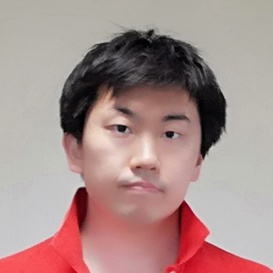atcoder

# # Problem

Given 4 integers $0 \geq A, B, C, D \geq 9$, put 3 add or sub operators.
Report a combination of them whose result is 7.

# # Time complexity

$O(1)$

# # Solution

string S;
char ops[] = { '-', '+' };

void input() {
cin >> S;
}

#define ctoi(x) (x - '0')

void solve() {
Int x = 0;
loop(first, 0, 2) {
loop(second, 0, 2) {
loop(third, 0, 2) {
x = ctoi(S);
if (first) x += ctoi(S);
else x -= ctoi(S);
if (second) x += ctoi(S);
else x -= ctoi(S);
if (third) x += ctoi(S);
else x -= ctoi(S);
if (x == 7) {
cout << S << ops[first] << S << ops[second] << S << ops[third] << S << "=7" << endl;
return;
}
}
}
}
cout << -1 << endl;
}

int main(void) {
input();
solve();
return 0;
}Remote freelancer. A web and mobile application enginner.
Traveling around the world based on East Asia.
I'm looking forward to your job offers from all over the world!

Offer jobs or contact me!